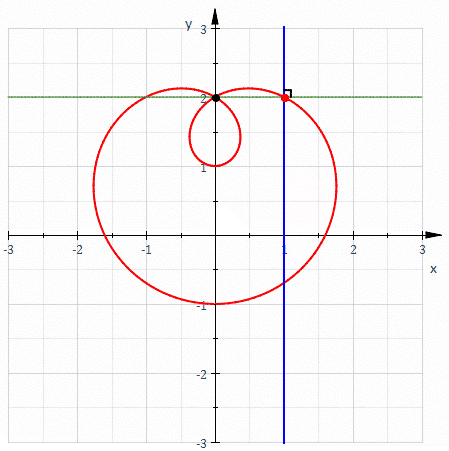# Negative pedal curveCircle — negative pedal curve of a limaçon

In geometry, a negative pedal curve is a plane curve that can be constructed from another plane curve C and a fixed point P on that curve. For each point X  P on the curve C, the negative pedal curve has a tangent that passes through X and is perpendicular to line XP. Constructing the negative pedal curve is the inverse operation to constructing a pedal curve.

## Definition

In the plane, for every point X other than P there is a unique line through X perpendicular to XP. For a given curve in the plane and a given fixed point P, called the pedal point, the negative pedal curve is the envelope of the lines XP for which X lies on the given curve.

## Parameterization

For a parametrically defined curve, its negative pedal curve with pedal point (0; 0) is defined as## Properties

The negative pedal curve of a pedal curve with the same pedal point is the original curve.